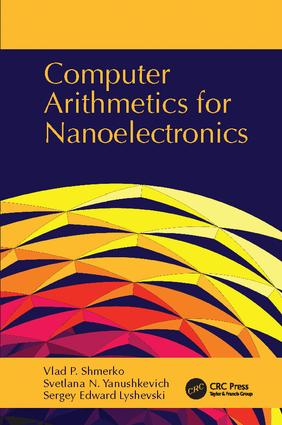# Computer Arithmetics for Nanoelectronics

## 1st Edition

CRC Press

780 pages | 440 B/W Illus.

##### Purchasing Options:\$ = USD
Paperback: 9781138113572
pub: 2017-06-14
\$82.95
x
Hardback: 9781420066210
pub: 2009-02-23
Currently out of stock
\$220.00
x

FREE Standard Shipping!

### Description

Emphasizes the Basic Principles of Computational Arithmetic and Computational Structure Design

Taking an interdisciplinary approach to the nanoscale generation of computer devices and systems, Computer Arithmetics for Nanoelectronics develops a consensus between computational properties provided by data structures and phenomenological properties of nano and molecular technology.

Covers All Stages of the Design Cycle, from Task Formulation to Molecular-Based Implementation

The book introduces the theoretical base and properties of various data structures, along with techniques for their manipulation, optimization, and implementation. It also assigns the computational properties of logic design data structures to 3D structures, furnishes information-theoretical measures and design aspects, and discusses the testability problem. The last chapter presents a nanoscale prospect for natural computing based on assorted computing paradigms from nature.

Balanced Coverage of State-of-the-Art Concepts, Techniques, and Practices

Up-to-date, comprehensive, and pragmatic in its approach, this text provides a unified overview of the relationship between the fundamentals of digital system design, computer architectures, and micro- and nanoelectronics.

Introduction

Computational paradigms for nanocomputing structures

Biological inspiration for computing

Self-assembly

Molecular computing devices

Fault tolerance

Computing in 3D

Multivalued processing

Computational Nanostructures

Introduction

Theoretical background

Analysis and synthesis

Implementation technologies

Predictable technologies

Nanoelectronic networks

Switch-based computing structures

Spatial computational nanostructures

Binary Arithmetic

Introduction

Positional numbers

Counting in a positional number system

Basic arithmetic operations in various number systems

Binary arithmetic

Conversion of numbers in various radices

Overflow

Implementation of binary arithmetic

Other binary codes

Further study

Residue Arithmetic

Introduction

Residue arithmetic

Further study

Graph-Based Data Structures

Introduction

Graphs in discrete device and system design

Basic definitions

Tree-like graphs and decision trees

Voronoi diagrams

Further study

Foundation of Boolean Data Structures

Introduction

Definition of algebra over the set {0, 1}

Boolean functions

Fundamentals of computing Boolean functions

Proving the validity of Boolean equations

Gates

Local transformations

Properties of switching functions

Further study

Boolean Data Structures

Introduction

Data structure types

Relationships between data structures

The truth table

K-map

Cube data structure

Graphical data structure for cube representation

Logic networks

Networks of threshold gates

Binary decision trees

Decision diagrams

Further study

Fundamental Expansions

Introduction

Shannon expansion

Shannon expansion for symmetric Boolean functions

Techniques for computing symmetric functions

The logic Taylor expansion

Graphical representation of the fundamental expansions

Further study

Arithmetic of the Polynomials

Introduction

Algebra of the polynomial forms

GF(2) algebra

Relationship between standard SOP and polynomial forms

Local transformations for EXOR expressions

Factorization of polynomials

Validity check for EXOR networks

Fixed and mixed polarity polynomial forms

Computing the coefficients of polynomial forms

Decision diagrams

Techniques for functional decision tree construction

Functional decision tree reduction

Further study

Optimization of Computational Structures

Introduction

Minterm and maxterm expansions

Optimization of Boolean functions in algebraic form

Implementing SOP expressions using logic gates

Minimization of Boolean functions using K-maps

Boolean function minimization using decision diagrams

Optimization of Boolean functions using decision trees

Decision diagrams for symmetric Boolean functions

Measurement of the efficiency of decision diagrams

Representation of multi-output Boolean functions

Embedding decision diagrams into lattice structures

Further study

Multivalued Data Structures

Introduction

Representation of multivalued functions

Multivalued logic

Galois fields GF(m)

Fault models based on the concept of change

Polynomial representations of multivalued logic functions

Polynomial representations using arithmetic operations

Fundamental expansions

Further study

Computational Networks

Introduction

Data transfer logic

Implementation of Boolean functions using multiplexers

Demultiplexers

Decoders

Implementation of switching functions using decoders

Encoders

Design examples

Design example: magnitude comparator

Design example: BCD adder

The verification problem

Decomposition

Further study

Sequential Logic Networks

Introduction

Physical phenomena and data storage

Basic principles

Data structures for sequential logic networks

Latches

Flip-flops

Registers

Counters

Sequential logic network design

Mealy and Moore models of sequential networks

Data structures for analysis of sequential networks

Analysis of sequential networks with various types of flip-flops

Techniques for the synthesis of sequential networks

Redesign

Further study

Memory Devices for Binary Data

Introduction

Programmable devices

Random-access memory

Memory expansion

Programmable logic

Field programmable gate arrays

Further study

Spatial Computing Structures

Introduction

The fundamental principles of 3D computing

Spatial structures

Hypercube data structure

Assembling of hypercubes

N-hypercube

Embedding a binary decision tree into an N-hypercube

Assembling N-hypercubes

Representation of N-hypercubes using H-tree

Spatial topological measurements

Linear Cellular Arrays

Introduction

Linear arrays based on systolic computing paradigm

Spatial systolic arrays

Linear arrays based on linear decision diagrams

Linear models of elementary functions

Logic networks and linear decision diagrams

Linear models for logic networks

Linear models for multivalued logic networks

Linear word-level representation of multivalued functions using logic operations

3D computing arrays design

Further study

Information and Data Structures

Introduction

Information-theoretical measures

Information-theoretical measures

Information measures of elementary switching function of two variables

Information-theoretical measures in decision trees

Information-theoretical measures in multivalued functions

Ternary and pseudo-ternary decision trees

Design for Testability

Introduction

Fault models

Controllability and observability

Functional decision diagrams for computing Boolean differences

Random testing

Design for testability techniques

Further study

Error Detection and Error Correction

Introduction

Channel models

The simplest error detecting network

Discrete memoryless channel

Linear block codes

Cyclic codes

Block codes

Arithmetic codes

Further study

Natural Computing

Introduction

Fundamentals of the intermediate data structures

Self-assembling

Fractal-based models for self-assembly

Logic network design using directed evolution

Neural-based computing

Further study

Index

### Subject Categories

##### BISAC Subject Codes/Headings:
TEC008010
TECHNOLOGY & ENGINEERING / Electronics / Circuits / General
TEC027000
TECHNOLOGY & ENGINEERING / Nanotechnology & MEMS